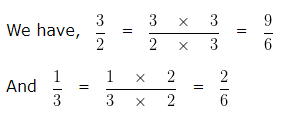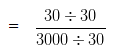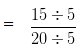# Ratio and Proportion Worksheets Grade 5Q.1) Compare the ratios 3 : 2 and 1 : 3 ?
a) 3 : 2 > 1 : 3
b) 3 : 2 < 1 : 3

Q.2) Divide ₹ 1200 between A and B in the ratio 3 : 5 ?
a) A = ₹ 750 , B = ₹ 450
b) A = ₹ 450 , B = ₹ 750
c) A = ₹ 700 , B = ₹ 500

Q.3) Find the missing terms: 18 : 20 : : _______ : 10 ?
a) 15
b) 12
c) 9

Q.4) Find the ratio of 30 g to 3 kg ?
a) 1 : 100
b) 100 : 1
c) 100 : 3

Q.5) Are 5 , 4 , 5 , 7 in proportion ?
a) No
b) Yes

Q.6) Hari can walk 12 km in 3 hours . How much can he walk in 8 hours , If he maintains the same speed all the time ?
a) 29 km
b) 34 km
c) 32 km

Q.7) If 6 oranges cost ₹ 36 . What is the cost of 5 oranges ?
a) ₹ 30
b) ₹ 33
c) ₹ 26

Q.8) Convert the ratio 15 : 20 in its simplest form ?
a) 3 : 4
b) 4 : 3
c) 5 : 3

Q.9) Write the ratio for the Fraction 6/7 ?
a) 6 : 5
b) 1 : 6
c) 6 : 7

Q.10) If the two numbers are in the ratio 4 : 3 and the sum of the two numbers is 56 . Find the two numbers ?
a) First number = 28 , Second number = 20
b) First number = 24 , Second number = 32
c) First number = 32 , Second number = 24

### Ratio and Proportion Worksheets Grade 5 Explanations

Q.1) Explanation – Ratio and Proportion Worksheets Grade 5

We can write
3 : 2 = 3/2 and 1 : 3 = 1/3
To find, Which Ratio is greater, we would need to convert the two Ratios into Like Fractions
LCM of Denominator 2 and 3 is 6
Making the Denominator of each Fraction equal to 6In case of Like Fractions, the Fraction whose numerator is greater is larger. Hence we can say 9/6 > 2/6
That is 3/2 > 1/3
Hence, 3 : 2 > 1 : 3

Correct Answer – a) 3 : 2 > 1 : 3

Q.2) Explanation – Ratio and Proportion Worksheets Grade 5

Total Sum of money = ₹ 1200
Given ratio = 3 : 5
Sum of ratio terms = ( 3 + 5 ) = 8
A would receive 3/8 part of ₹ 1200
B would receive 5/8 part of ₹ 1200
That is,
A ‘s share = ₹ ( 1200 x 3/8 ) = ₹ 450
B ‘s share = ₹ ( 1200 x 5/8 ) = ₹ 750

Correct Answer – b) A = ₹ 450 , B = ₹ 750

Q.3) Explanation – Ratio and Proportion Worksheets Grade 5

Let the missing term be y
Then we can say
18/20 = y/10
On cross multiplication we get,
18 x 10 = y x 20
180 = 20 y
20 y = 180
y = 180/20
y = 9
So, the missing number is 9

Q.4) Explanation – Ratio and Proportion Worksheets Grade 5

To find the ratio of two Quantities, we need to convert them into same Unit.
Converting both the Quantities in the same unit,
Since, 1 kg = 1000 g
3 kg = 3 x 1000 g
= 3000 g
Now the ratio becomes, 30 g : 3000 g
Or 30/3000
Now,
Dividing 30 and 3000 by their H.C.F 30
The given fraction would be= 1/100
Therefore, the required ratio is 1 : 100

Correct Answer – a) 1 : 100

Q.5) Explanation – Ratio and Proportion Worksheets Grade 5

If four numbers are in proportion, then the
Product of Means of the numbers = Product of Extremes of the numbers
Here, Means are 4 and 5
Extremes are 5 and 7
Product of Extremes = 4 x 5 = 20
Product of Means = 5 x 7 = 35
Since, Product of Extremes ≠ Product of Means
Hence, 5 , 4 , 5 , 7 are not in proportion .

Q.6) Explanation – Ratio and Proportion Worksheets Grade 5

Distance covered by Hari in 3 hours = 12 km
In unitary method first of all we find the value of unit quantity and then find the value of given quantity
Distance covered by Hari in 1 hours = 12/3 km ( Lower the time, Lower the distance )
Now,
Distance covered by Hari in 8 hours = ( 12/3 x 8 ) km ( Higher the time, Higher the distacne )
= 32 km
Therefore, the Distance covered by Hari in 8 hours is 32 km

Correct Answer – c) 32 km

Q.7) Explanation – Ratio and Proportion Worksheets Grade 5

Cost of 6 oranges = ₹ 36
In unitary method first of all we find the value of unit quantity and then find the value of given quantity
Cost of 1 orange = ₹ 36/6 ( Less Quantity, Less Cost )
Now,
Cost of 5 oranges = ₹ ( 36/6 x 5 ) ( More Quantity, More Cost )
= ₹ 30
Therefore, the cost of 5 oranges is ₹ 30

Correct Answer – a) ₹ 30

Q.8) Explanation – Ratio and Proportion Worksheets Grade 5

Ratio = 15 : 20
Ratio can be reduced to its Simplest form by Dividing the two terms by their H.C.F.
H.C.F of 15 and 20 is 5
Now, Dividing 15 and 20 by their H.C.F 5= 3/4
Therefore, the Simplest form of 15 : 20 is 3 : 4

Correct Answer – a) 3 : 4

Q.9) Explanation – Ratio and Proportion Worksheets Grade 5

Given Fraction = 6/7
If any two numbers “a” and “b”are written as a Fraction a/b and cannot be further simplified, then the ratio for the Fraction can be written as
a : b ( : represents the sign of Ratio )
On comparing the given Fraction with a/b
We get
a = 6 and b = 7
6 : 7
Hence, ratio of the Fraction 6/7 is 6 : 7

Correct Answer – c) 6 : 7

Q.10) Explanation – Ratio and Proportion Worksheets Grade 5

Given Ratio of two numbers is = 4 : 3
Sum of the numbers is = 56
Sum of ratio terms = ( 4 + 3 )
= 7
First number = 4/7 of Sum of the given numbers
Second number = 3/7 of Sum of the given numbers
Therefore,
First number = 4/7 x 56 = 32
Second number = 3/7 x 56 = 24
So, the two numbers are 32 , 24

Correct Answer – c) First number = 32 , Second number = 24